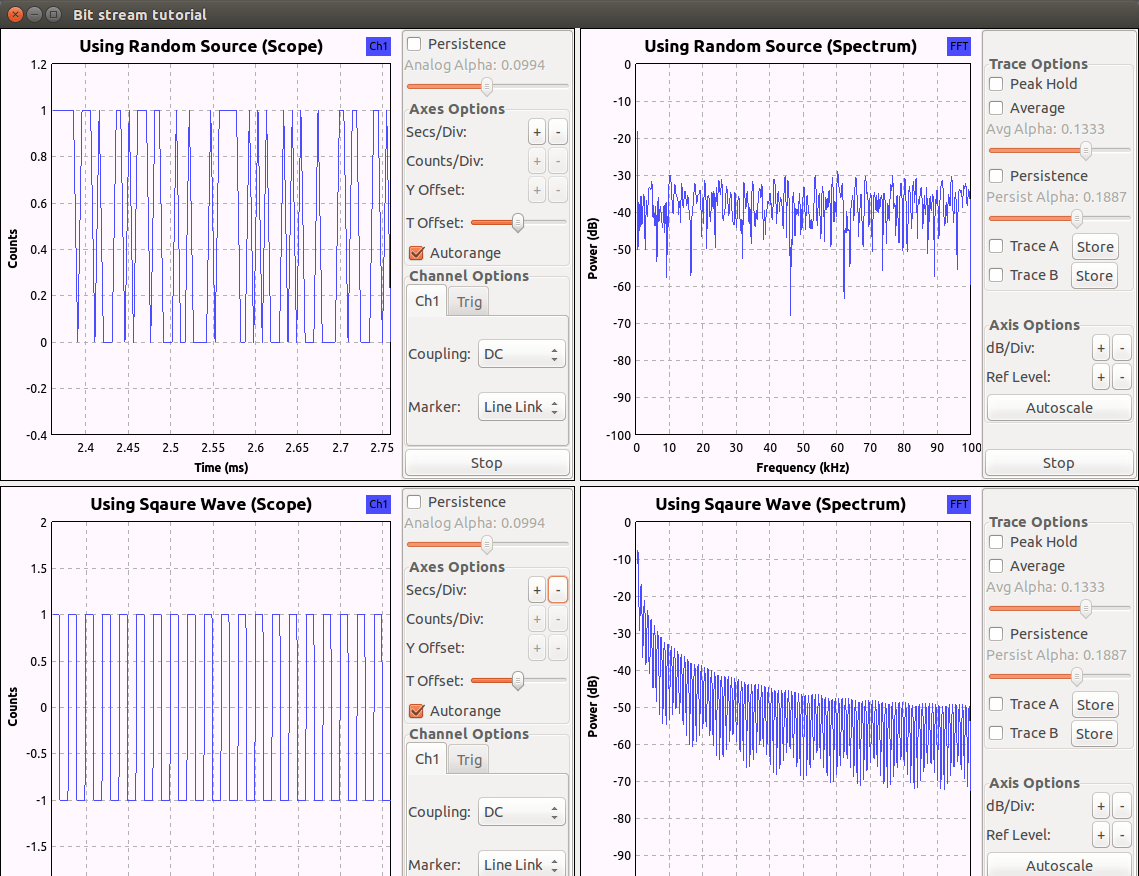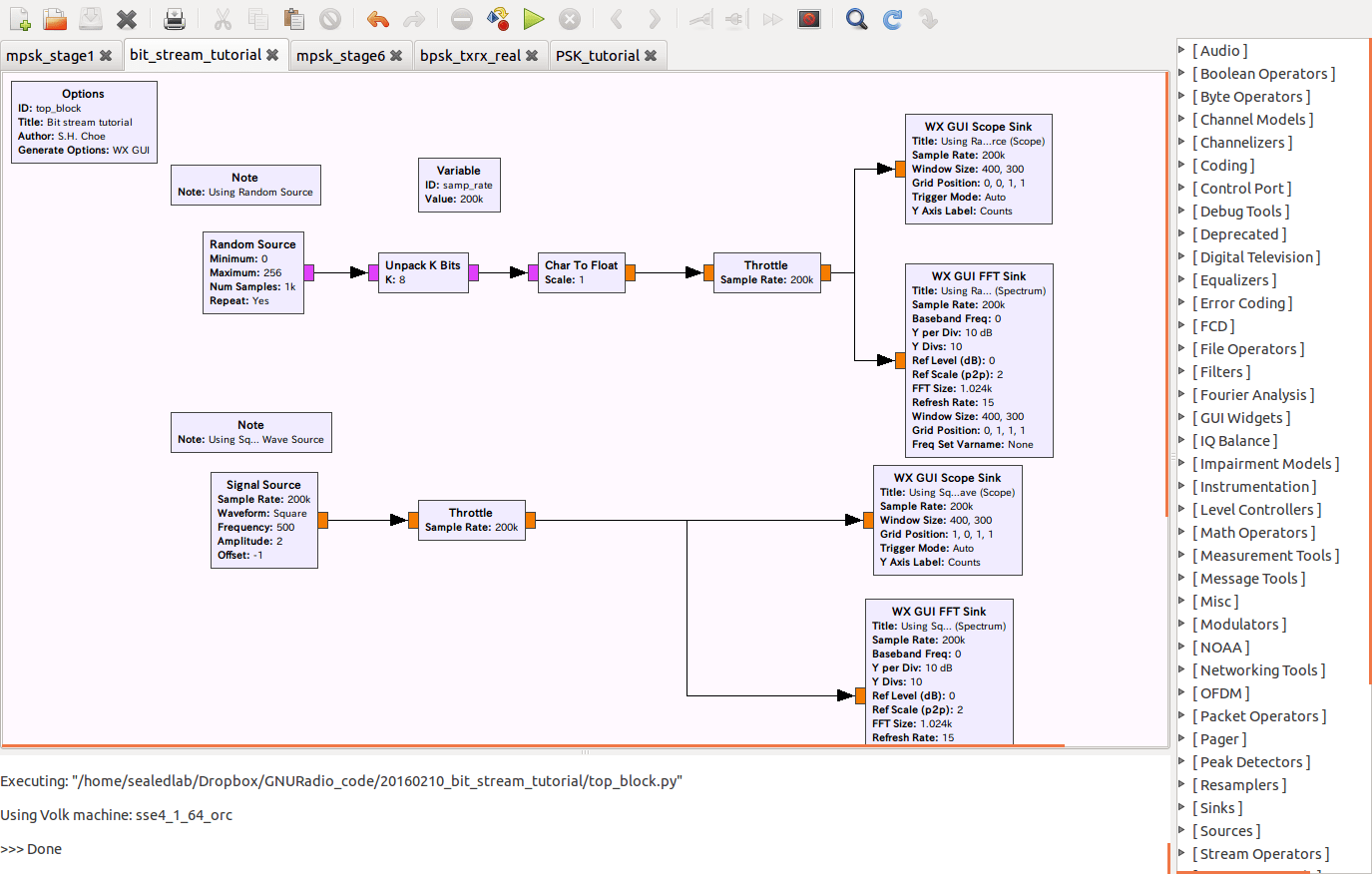We will see how to make bit stream in GNU Radio.

Generally speaking, we can two different methods. One is using the Random noise block and the other is the using the Signal source block.

First, we make a flow diagram like a following picture.

bit_stream_tutorial.grc(1) Random source block

First we are making 8 bit random signal (Maximum 256 = 2^8) and the output is defined as Byte.

The output signal will be unpacked data stream by Unpack K bits block. K should be 8 here.

and Char to Float block will convert the data stream from Byte to Float.

(2) Signal source block

This is very simple methods. We are just using the square wave. Please change the amplitude into 2 and offset into -1. Then you will see the 0 and 1 bit stream soon.

Here is the results of the grc file. Please do not miss the spectrum plot of each method. The spectrum of the random source looks flat over the frequency whereas the peak is observed in the middle frequency from the square wave source.## 1件のコメント

1.Abdul Samad Usman

Is there any way to store this bit stream into a file so that it could be read later on. I tried file sink but the output file gets corrupted. I want simple 1s and 0s written in a text file

このサイトはスパムを低減するために Akismet を使っています。コメントデータの処理方法の詳細はこちらをご覧ください